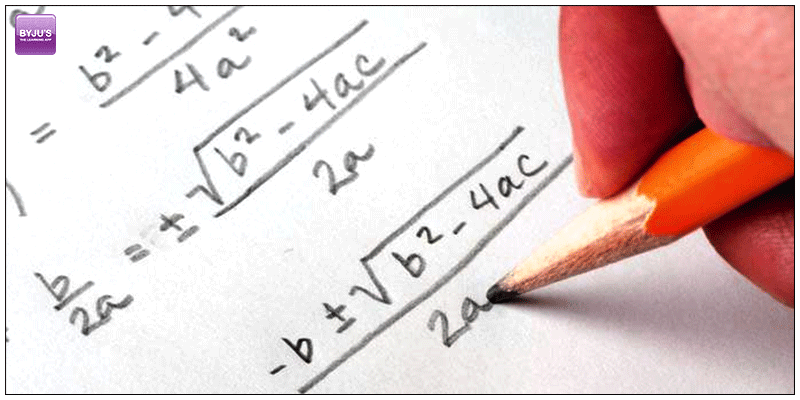# Algebra Preparation Tips for GMATIt is true, in GMAT Exam, Quant offers you the maximum chances of  securing highest scores. But we should also remember that it gives enormous mental trauma for the GMAT aspirants. Especially to those aspirants who are detached from mathematics for more than couple of years and mathematics haunt them like nightmare.

Algebra is the  topic we started learning since we’re in 6th standard or in 7th standard. It is a combination of numbers and variables and we’re supposed to find the value of the variables. And in examination hall, these variables shook our mind and trembles our performance.

We are giving you five cool tips to tackle the algebraic expression with less tension:

#cooltip 1: Understand first:

The first tip from us is to before solving a specific problem understand the equation first. Take few breathe first, before initiating to solve the equation.

#cooltip 2: Don’t mug-up:

You should understand that, GMAT is unlikely to school exams where you can mug-up a specific question and solve in the test and acquire good marks. But GMAT is a game of tricks and techniques if want to hit the score of 40+ out of 70 in Quant section.

#cooltip 3: Learn the Algebra identities first:

Algebra Identities:

Difference of Squares

• $a^{2} – b^{2} = (a+b)(a-b)$

Difference of Cubes:

• $a^{3} – b^{3} = (a-b)(a^{2} + ab + b^{2})$

Sum of cubes:

• $a^{3} + b^{3} = (a+b)(a^{2} – ab + b^{2})$

Special Algebra Expansion:

Formula for $(a + b)^{2} \; and \; (a – b)^{2}$

• $(a + b)^{2} = a^{2} + 2ab + b^{2}$
• $(a – b)^{2} = a^{2} – 2ab + b^{2}$

Formula for $(a + b)^{3} \; and \; (a – b)^{3}$

• $(a + b)^{3} = a^{3} + 3a^{2}b + 3ab^{2} + b^{3}$
• $(a – b)^{3} = a^{3} – 3a^{2}b + 3ab^{2} – b^{3}$

Formula

• $ax^{2} + bx + c = 0$

Where a, b and c are the leading coefficients.

The roots for this quadratic equation will be:

$\large x = \frac{-b \pm \sqrt{b^{2} – 4ac}}{2a}$

## Arithmetic Progression

Discuss the following arithmetic progression:

• $a + (a + d) + (a + 2d) + (a + 3d) + …$

Where:

• a is the initial term
• d is the common difference

The nth term

The $n^{th}$ term, $T_{n}$ of the arithmetic progression is:

• $T_{n} = a + (n-1)d$

Sum of the first n term

The sum of the first n terms of the arithmetic progression is:

Geometric Expression:

Discuss the following geometric progression:

• $a + ar + ar^{2} + ar^{3} + …$

Where:

• a is the scale factor
• r is the common ratio

The nth term

The $n^{th}$ term, $T_{n}$ of the geometric progression is:

• $T_{n} = ar^{n -1}$

Sum of the first n terms

The sum of the first n terms, $S_{n}$ is:

The sum to infinity

If -1 < r < 1, the sum to infinity, $S_{\infty}$ is:

#cooltip 4: Practice… Practic … & Practice:

All of us heard about the proverb “Practice makes a man perfect”, so it’s true in this context, the more you practice the more you’ll be able to grasp the tricks and techniques. And it will increase your solving speed.

#cooltip 5: Be calm while solving:

While solving, the equation focus on the problem with a cool mind. If you pamper it will increase your stress level and knowing the method of solving you might go wrong due to it.

To learn more and to grow more give us a missed call or SMS on +918884544444. Reach us through email byjusforgmat@gmail.com.